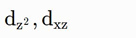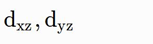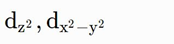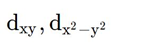# NEET Chemistry Question Paper 2016 with Answers

46. Which one of the following compounds shows the presence of intramolecular hydrogen bond?

(A) H2O2     (B) HCN

(C) Cellulose

(D) Concentrated acetic acid

Chemistry Notes

Physics Notes

Biology Notes

47. The molar conductivity of a 0.5 mol dm-3 solution of AgNO3 with electrolytic conductivity of 5.76 x10-3S cm-1 at 298 K

(A) 2.88 S cm2/mol

(B) 11.52 S cm2/mol

(C) 0.086 S cm2/mol

(D) 28.8 S cm2/mol

48. The decomposition of phosphine (PH3) on tungsten at low pressure is a first-order reaction. It is because the

(A) Rate is proportional to the surface coverage

(B) Rate is inversely proportional to the surface coverage

(C) Rate is independent of the surface coverage

(D) Rate of decomposition is very slow

49. The coagulation values in millimoles per litre of the electrolytes used for the coagulation of As2S3 are given below :

I. (NaCl) = 52        II. (BaCl2) = 0.69        III. (MgSO4) = 0.22

The correct order of their coagulating power is

(A) I > II > III              (B) II > I > III

(C) III > II > I              (D) III > I > II

50. During the electrolysis of molten sodium chloride, the time required to produce 0.10 mol of chlorine gas using a current of 3 amperes is

(A) 55 minutes

(B) 110 minutes

(C) 220 minutes

(D) 330 minutes

51. How many electrons can fit in the orbital for which n = 3 and l = 1?

(A) 2

(B) 6

(C) 10

(D) 14

Chemistry Notes

Physics Notes

Biology Notes

52. For a sample of perfect gas when its pressure is changed isothermally from pi to pf, the entropy change is given by

(A) ΔS = nR ln(pf / pi)

(B) ΔS = nR ln(pi / pf)

(C) ΔS = nRT ln(pf / pi)

(D) ΔS = RT ln(pi / pf)

53. The van’t Hoff factor (i) for a dilute aqueous solution of the strong electrolyte barium hydroxide is

(A) 0

(B) 1

(C) 2

(D) 3

54. The percentage of pyridine C5H5N that forms pyridinium ion C5H5N+H in a 0.10 M aqueous pyridine solution (Kb for C5H5N = 1.7 x 10-9) is

(A) 0.0060%

(B) 0.013%

(C) 0.77%

(D) 1.6%

54. The percentage of pyridine C5H5N that forms pyridinium ion C5H5N+H in a 0.10 M aqueous pyridine solution (Kb for C5H5N = 1.7 x 10-9) is

(A) 0.0060%

(B) 0.013%

(C) 0.77%

(D) 1.6%

56. If the E°cell for a given reaction has a negative value, which of the following gives the correct relationships for the values of ΔG° and Keq?

(A) ΔG° > 0; Keq < 1

(B) ΔG° > 0; Keq < 1

(C) ΔG° > 0; Keq < 1

(D) ΔG° > 0; Keq < 1

57. Which one of the following is incorrect for ideal solution?

(A) ΔHmix  = 0

(B) ΔUmix = 0

(C) ΔP = Oobs – Pcalculated by raiul’s law = 0

(D) ΔGmix = 0

58. The solubility of AgCl(s) with solubility product 1.6 x 10-10 in 0.1 M NaCl solution would be

(A) 1.26 x 10-5 M

(B) 1.6 x 109 M

(C) 1.6 x 10-11 M

(D) Zero

Chemistry Notes

Physics Notes

Biology Notes

59. Suppose the elements X and Y combine to form two compounds XY2 and X3Y2. When 0.1 mole of XY2 weighs 10 g and 0.05 mole of X3Y2 weighs 9 g, the atomic weights of X and Y are

(A) 40, 30

(B) 60, 40

(C) 20, 30

(D) 30, 20

60. The number of electrons delivered at the cathode during electrolysis by a current of 1 ampere in 60 seconds is (charge on electron = 1.60 x 10-19 C)

(A) 6 x 1023

(B) 6 x 1020

(C) 3.75 x 1020

(D) 7.48 x 1023

61. Boric acid is an acid because its molecule

(A) Contains replaceable H+ ion

(B) Gives up a proton

(C) Accepts OHfrom water releasing proton

(D) Combines with proton from water molecule

62. AlF3 is soluble in HF only in presence of KF. It is due to the formation of

(A) K3[AlF3H3]

(B) K3[AlF6]

(C) AlH3

(D) K[Al3H]

63. Zinc can be coated on iron to produce galvanized iron but the reverse is not possible. It is because

(A) Zinc is lighter than iron

(B) Zinc has lower melting point than iron

(C) Zinc has lower negative electrode potential than iron

(D) Zinc has higher negative electrode potential than iron

Chemistry Notes

Physics Notes

Biology Notes

64. The suspension of slaked lime in water is known as

(A) Limewater

(B) Quicklime

(C) Milk of lime

(D) Aqueous solution of slaked lime

65. The hybridizations of atomic orbitals of nitrogen in NO2+,NO3- and NH4+ respectively are

(A) sp, sp3 and sp2

(B) sp2, sp3 and sp

(C) sp, sp2 and sp3

(D) sp2, sp and sp3

66. Which of the following fluoro – compounds is most likely to behave as a Lewis base?

(A) BF3

(B) PF3

(C) CF4

(D) Si4

67. Which of the following pairs of ions is isoelectronic and isostructural?

(A) CO32-, NO3

(B) Cl3, CO32-

(C) SO32-, NO3

(D) ClO2, SO32-

68. In context with beryllium which one of the following statements is incorrect

(A) It is rendered passive by nitric acid

(B) It form Be2C

(C) Its salts rarely hydrolyze

(D) Its hydride is electron – deficient and polymeric

69. Hot concentrated sulphuric acid is a moderately strong oxidizing agent. Which of the following reactions does not show oxidizing behaviour?

(A) Cu + 2H2SO4 → CuSO4 + SO2 + 2H2O

(B) 3S + 2H2SO4 → 3SO2 + 2H2O

(C) C + 2 H2SO4 → CO2 + 2SO2 + 2H2O

(D) Ca2 + H2SO4 → CaSO4 + 2HF

70. Which of the following pairs of d – orbitals will have electron density along the axes?

(A)(B)(C)(D)71. The correct geometry and hybridization for XeF4 are

(A) Octahedral, sp3z2

(B) Trigonal bipyramidal, sp3d

(C) Planar triangle, sp3d3

(D) Square planar, sp3d2

72. Among the following, which one is a wrong statement ?

(A) PH5 and BiCl5 do not exist

(B) p bonds are present in SO2

(C) SeF4 and CH4 have same shape

(D) I3+ has bent geometry